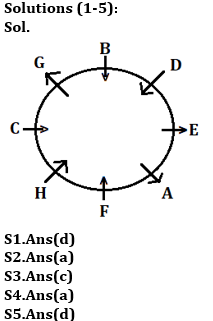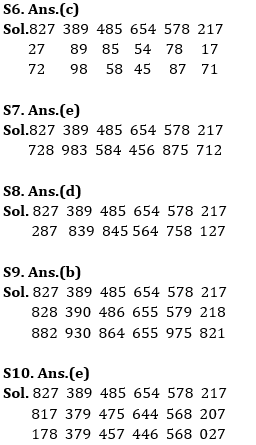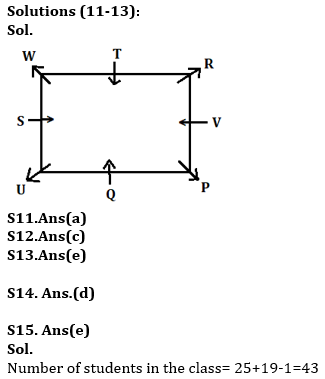Latest Banking jobs   »   Reasoning Ability Quiz For ESIC- UDC,...

# Reasoning Ability Quiz For ESIC- UDC, Steno, MTS Prelims 2022- 24th January

Directions (1-5): Study the information carefully and answer the questions given below.
Eight persons A, B, C, D, E, F, G and H are sitting in a circular table. Some of them face inside and some of them face outside. All information is not necessarily in same order.
B faces F. E sits immediate left of A. Two persons sit between B and H. C sits immediate left of H. G sits second to the right of D. D sits third to the left of C. G doesn’t sit opposite to the person who sits immediate right of B. A faces same direction as E and G. F is not immediate neighbor of E. Immediate neighbor of A face opposite direction to each other.

Q1. Who among the following sits second to the right of E?
(a) The one who sits immediate left of D
(b) C
(c) H
(d) The one who sits second to right of C
(e) None of these

Q2. How many persons sit between C and A when counted from left of A?
(a) Four
(b) Two
(c) More than four
(d) Three
(e) None

Q3. Who among the following sits third to the right of B?
(a) G
(b) E
(c) H
(d) C
(e) None of these

Q4. Which of the following sits third to left of G?
(a) F
(b) A
(c) E
(d) D
(e) None of these

Q5. Four of the following five are alike in certain way based from a group, find the one that does not belong to that group?
(a) H
(b) F
(c) B
(d) E
(e) C

Directions (6-10): These questions are based on the following six numbers.

827   389   485   654   578   217

Q6. If two digit numbers are formed by taking the 2nd and 3rd digits respectively of each of these numbers and then the two digits of each of these two digit numbers are interchanged, then which number from the given numbers will be the second highest number in the revised form?
(a) 389
(b) 485
(c) 578
(d) 827
(e) None of these

Q7. If these numbers are written with their digits in the reverse order, which number among them will be third highest?
(a) 485
(b) 578
(c) 217
(d) 389
(e) 827

Q8. If the first two digits of each number are interchanged, which among these numbers will be the 2nd lowest number?
(a) 485
(b) 645
(c) 217
(d) 827
(e) None of these

Q9. If one is added to each of the given numbers and then each digit is arranged in descending order within each number, then which of the following number is smallest?
(a) 485
(b) 654
(c) 578
(d) 217
(e) 827

Q10. If one is subtracted from middle digit in each given numbers and then all the digits in each number is arranged in ascending order then which of the following number is second smallest?
(a) 485
(b) 654
(c) 578
(d) 217
(e) 827

Directions (11-13): Study the information carefully and answer the questions given below.
Eight persons i.e. P, Q, R, S, T, U, V and W are sitting in a square table in such a way that the Four persons sit at four corner and face outside and four persons who sit at middle of the side of square table face inside. S sits third to the right of P. T sits second to left of S. W sits second to left of R. Only one person sits between Q and V. W face outside. The person who faces Q sits third to the right of U.

Q11. Who among the following sits immediate left of U?
(a) Q
(b) S
(c) T
(d) V
(e) None of these

Q12. How many persons sit between W and Q, when counted to the right of Q?
(a) Two
(b) Three
(c) More than Three
(d) One
(e) None

Q13. Who among the following sits third to the left of R?
(a) Q
(b) T
(c) W
(d) P
(e) None of these

Q14. In a certain code, FINANCIAL is written as ZMRUMOZRX. How is INTERVIEW written in the code?
(a) VGMRGDVRE
(b) RIVGMDVRE
(c) VGIDVREMR
(d) VGMRIDVRE
(e) None of these

Q15. Rahul ranked 25th from the top and 19th from the bottom in a class. How many students are there in the class?
(a) 44
(b) 40
(c) 33
(d) 35
(e) None of these

Solutions#### Congratulations!